# Random RMS Calculator Tutorial

#### Random,Test Generation

Compute random acceleration, velocity, and displacement values from a breakpoint table.

A random spectrum is defined as a set of frequency and amplitude breakpoints. Consider the values on the table below:

 Frequency (Hz) Amplitude 20 0.01005 80 0.04000 350 0.04000 2000 0.00704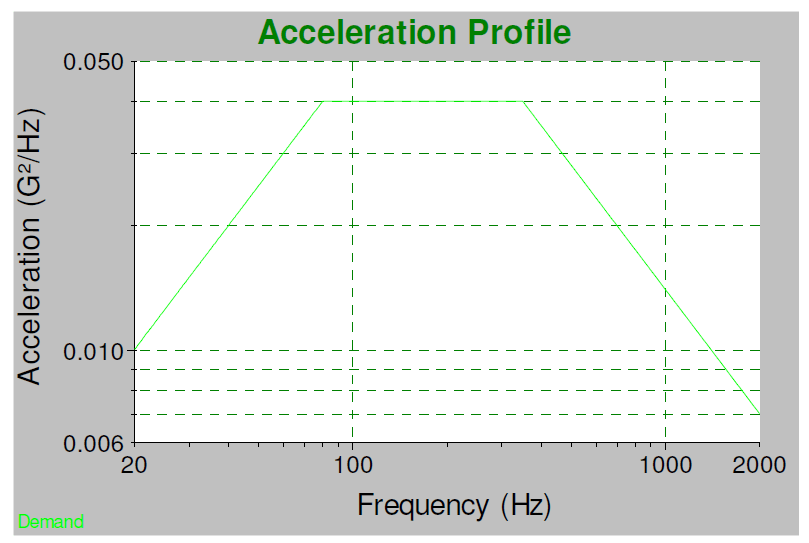To compute the root mean square (RMS) from the breakpoint values, we must calculate the area under the curve defined by the breakpoint values. At first, this may seem simple because it can be divided into a group of squares and triangles. However, the triangles are the result of straight lines on log-log graph paper, not linear. We can still take advantage of the triangle shapes but need to use a specific formula to compute the area of triangles on log-log graph paper.

The definition of a straight line on log-log graphs between two breakpoints (f1,a1) and (f2,a2) is a power relationship, where the slope is the exponent, and the offset is the multiplicative factor.

 (1)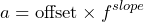(1)

The slope and offset that define the straight line are computed as follows:

 (2)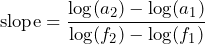(2) (3)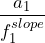(3)

Given the slope and offset, we can integrate from f1 to f2 to compute the area under the line:

 (4)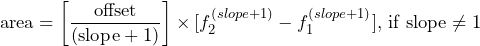(4)

However, this formula does not hold if the slope equals 1. In this case, we note that a = offset / f, which integrates into a natural log function.

Hint: some programs such as Microsoft ExcelTM define the log() function as a base-10 logarithm and the ln() function as the natural (base-e) logarithm. Be sure to use the correct function in your calculation. As a test, log(2.71828182845905) = 1.0 for a natural logarithm.

 (5)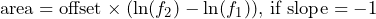(5)

We can use Equations (4) or (5) to calculate the area under the curve for each pair of breakpoints. The total area is the sum of the individual area calculations between each breakpoint pair and is the mean-square acceleration. The square root of the result equates to the RMS acceleration level. If we use the example breakpoints, the sum is computed as follows:

 Frequency (Hz) Amplitude (G2/Hz) Slope Offset Area (G2) 20 0.01005 80 0.04000 0.9964 0.000508 1.50 350 0.04000 0 0.04000 10.80 2000 0.00704 -0.9967 13.73 24.47 Total 36.77 G2
 (6)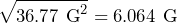The sum of the area values equates to a mean-square acceleration of 36.77 G2. The square root of this value gives an overall RMS value of 6.064 G RMS. The acceleration units are the square root of the acceleration density units. For a density unit of (m/s2)2/Hz, the result will have a unit of m/s2. For a density unit of m2/s3 (a reduced form of (m/s2)2/Hz), the result will have a unit of m/s2.

The RMS velocity can be computed the same as acceleration; however, the breakpoint values must be converted from units of acceleration-squared/Hz to velocity-squared/Hz with appropriate unit conversion if required. This conversion is performed with Equation (6), which defines the relationship between velocity and acceleration for a sine wave of a given frequency.

 (7)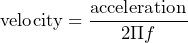(6)

As a result, the equation in velocity density for the lines connecting the breakpoints becomes:

 (8)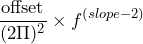(7)

This can be integrated from f1 to f2 to equate the area under the velocity line.

 (9)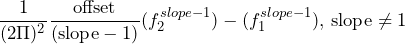(8)

When slope = 1, we must use a natural log function.

 (10)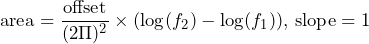(9)

Then, we can sum the areas to calculate the mean-square velocity, and take the square root of the value to get RMS velocity for the random spectrum. When using acceleration unit G, we must also apply a conversion factor to get a suitable velocity unit. Common conversions are G to inches/s2 or m/s2.

 (11)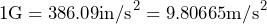(10)

Using the previous example, the RMS velocity is computed as follows:

 Frequency (Hz) Amplitude (G2/Hz) Slope Offset Area ((Gs)2) 20 0.01005 80 0.04000 0.9964 0.000508 1.760e-5 350 0.04000 0 0.04000 0.977e-5 2000 0.00704 -0.9967 13.73 0.141e-5 Total 2.878e-5

(12)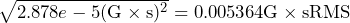Applying the unit conversion, we get:

(13)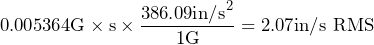The RMS displacement can also be computed the same as acceleration; however, the breakpoint numbers must be converted from units of acceleration-squared/Hz to displacement-squared/Hz with appropriate unit conversion if required. This conversion is performed with Equation (11), which defines the relationship between acceleration and displacement for a sine wave of a given frequency.

 (14)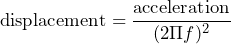(11)

As a result, the equation for the lines connecting the breakpoints in displacement density becomes:

 (15)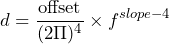(12)

Now, we can integrate this from f1 to f2 to get the area under the displacement line.

 (16)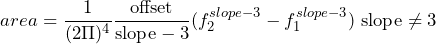(13)

When slope = 3, we need to use a natural log function:

 (17)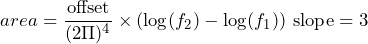(14)

Then, we can sum the areas to get the mean-square displacement and take the square root of the value to get RMS displacement for the random spectrum. When using acceleration units in G, you also need to apply a conversion factor such as Equation (10) to get a suitable displacement unit.

Using the previous example, the RMS displacement is computed as follows:

 Frequency (Hz) Amplitude (G2/Hz) Slope Offset Area ((Gs2)2) 20 0.01005 80 0.04000 0.9964 0.000508 3.773e-10 350 0.04000 0 0.04000 0.165e-10 2000 0.00704 -0.9967 13.73 0.0015e-10 Total 3.994e-10
 (18)After applying the unit conversion, we get:

(19)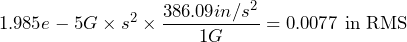However, we are more likely to be interested in peak-to-peak displacement rather than RMS displacement because shaker travel limits are rated as a peak-to-peak value. The vibration is Gaussian random, so it is not possible to find an absolute peak value. Still, we can compute an average or typical peak value. For Gaussian random values, the average one-sided peak level is about three times the RMS value (also called the 3-sigma level). To get double-sided displacement—e.g., peak-to-peak displacement—this number is doubled.

The typical peak-to-peak value is computed as:

Typical peak-peak displacement = 2 · 3 · (0.0077 in RMS) = 0.046 in peak-to-peak

It is important to understand that this value is the typical peak-to-peak displacement value and will occur relatively frequently during the test. However, you will also have occasional peaks at levels higher than 3·RMS. These peak heights can only be defined in terms of probabilities. Therefore, the appropriate question is not how high the peaks are but how often they will reach that height.

For Gaussian random data, the amplitudes will exceed 3·RMS 0.27% of the time and 4·RMS 0.006% of the time. There is a 0.0000002% chance that a peak will exceed 6·RMS. This probability is small but not impossible. In practice, 30% to 50% of the headroom should be above the typical peak-to-peak displacement value to account for the occasional higher peak levels.

Sine-on-Random tests have background random vibration with one or more sine tones overtop. The background random levels are computed the same as standard random as described above. The sine tones add to the random in the mean-square. We must sum all the squared values of the RMS levels, then take the square root of the result to calculate the overall RMS value. The sine tones are normally defined by their peak acceleration, so they must be converted from peak to RMS. For a sine tone, this is as simple as dividing the peak value by the square root of 2.

#### Sine-on-Random Example

Using the background random as defined above and add sine tones of 1.0G peak at 50Hz, 2.0G peak at 80Hz, and 1.5G peak at 110Hz.

 Frequency (Hz) Acceleration  G Peak Acceleration  G RMS Squared  G2 Random – 6.064 36.77 50 1.0 0.707 0.50 80 2.0 1.414 2.00 110 1.5 1.061 1.13 Total 40.40

(20)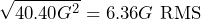Velocity functions the same way. Convert the acceleration to velocity using Equation (6), then convert the result to the appropriate velocity unit. When summing the squared values, the units for the background random and the sine tones should match.

 Frequency (Hz) Acceleration  G Peak Acceleration  G RMS Velocity  Gs RMS Squared  (Gs)2 random – – 2.878e-5 50 1.0 0.707 0.005364 0.507e-5 80 2.0 1.414 0.002251 0.792e-5 110 1.5 1.061 0.002813 0.236e-5 0.001535 Total 4.411e-5

(21)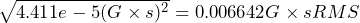Applying the unit conversion, we get:

(22)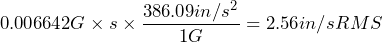Displacement would function the same way if we were interested in RMS values. However, to get peak displacement, the peak-to-peak sine displacement would be 2 times the square root of 2 multiplied by the RMS displacement for the sine tones. The RMS-to-(peak-to-peak) conversion factor for 3-sigma random peaks (as assumed for the random background vibration) would not apply to the sine tones.

To meet the overall peak-to-peak displacement requirements, it is better to assume that the peaks for the sine tones and the random background could occur at the same point in time. As such, the peak displacement values are simply added together.

To get the overall sine-on-random peak-to-peak displacement requirement, add the peak-to-peak displacements for the random background and each of the sine tones. Convert acceleration to displacement using Equation (11), then convert the displacement units using Equation (10).

 Frequency (Hz) Acceleration  (G Pk) Displacement  (Gs2 pk-pk) Displacement  in pk-pk random – – 0.046 50 1.0 1.433e-5 0.006 80 2.0 0.112e-5 0.004 110 1.5 0.444e-5 0.002 Total 0.058 in peak-to-peak